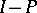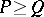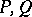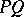# Projector

(diff) ← Older revision | Latest revision (diff) | Newer revision → (diff)

projection operator

A linear operatoron a vector spacesuch that.

Ifis a projection, so is, and together they define a direct sum decomposition. Conversely, a direct sum decomposition defines a projection. In Banach space theory a projection is usually also required to be bounded. Given a setof commuting projections, there is a partial order on, defined byif and only if. The intersection and union of two commuting projectionsare, respectively, the projectionsand. A Boolean algebra of projections is a set of commuting projections containing the zero and identity operations and which is closed under intersection of projections (i.e., taking the greatest lower bound) and union of projections (i.e., taking the least upper bound). Such Boolean algebras of projections play an important role in (self-adjoint and spectral) operator theory, cf. Spectral measure and [a1].